# Quick Tip: Collision Reaction Between a Circle and a Line Segment

In the previous Quick Tips, we've looked at collision detection: essentially, detecting that two shapes have overlapped. Now, we're ready to look at collision reaction: making something happen due to a collision. In this Quick Tip, we'll look at the reactions of reflection and sliding.

## Final Result Preview

Let's look at the end result we'll achieve at the end of this tutorial. Each Flash demo has a restart button; click it to reset the position of the circles at the top of stage.

The first demo shows off reflection:

The second shows sliding:

## Step 1: The Reflection Formula

I've run through this topic several rounds with students, and experience has taught me that the head-on approach of explaining vector math to freshers results in blank faces and confused minds. So instead of putting up a Math lecture here, I shall refer those who are interested in investigating this topic further to Wolfram's page on reflection.

Here, I shall simplify my explanations with diagrams below. Recall vector addition: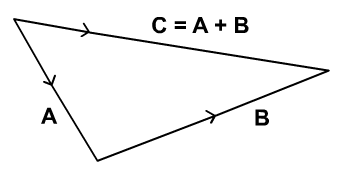Now, observe the diagram below. A is the circle's velocity before a collision, and A' is its velocity after the collision.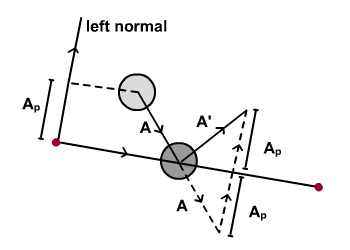It's obvious that A' = A + 2 V(Ap), where V(Ap) represents the vector with a magnitude of Ap, in the direction of the left normal. (You can see this by following the dashed lines.)

In order to obtain V(Ap), we shall project A onto the left normal.

## Step 2: Implementation

Here comes the ActionScript implementation of reflection. I've highlighted the important parts. Line 67 - 69 is to calculate V(Ap) (v_leftNormSeg2) and line 70 implements the formula. You may refer to the full Actionscript under Reaction1.as.

(You should recognise most of the code from the previous Quick Tip.)

## Step 3: An Interactive Version

Take note that this reflection formula is applicable to line of any gradient. In fact, you can program your line to be adjustable at runtime and see it reflecting circles like the Flash presentation below. Just click and drag near the lower end of the to redefine it.

## Step 4: Sliding Along Line

The concept of sliding along the line is almost identical to reflection. Observe the diagram below.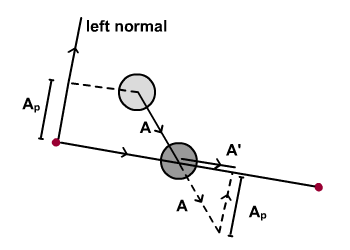The vector of slide is A' = A + V(Ap) with V(Ap) representing a vector with magnitude of Ap. Again, to obtain Ap we shall project A onto the left normal.

Note that as the circle is sliding along the line, it is colliding with the line. Of course, collision points differ among circles that collide onto line, so some overlap the line as they move along it. This doesn't look good, so we'll have to reposition them.

## Step 5: Redefine Location

Now, let's reposition circles on the line while maintaining their contact with line. Refer to the diagram below.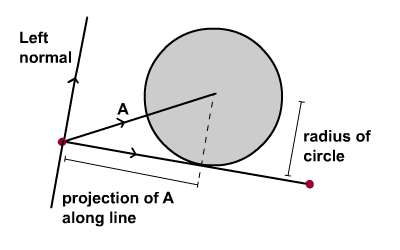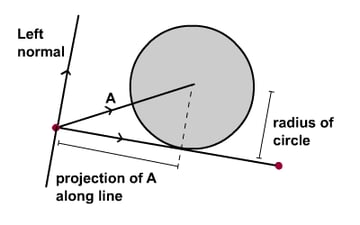An important variable to calculate is the projection of A along line. The radius of circle is readily available, and we already have B, so we can form the vectors of B and C. Adding the two will give us A, the exact location to reposition circle. Simple!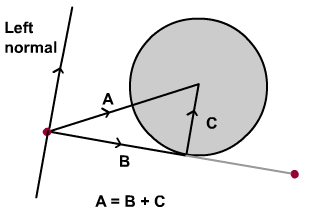The Flash presentation below is coded according to the mentioned idea. But there is one problem: the circles jitter along the line.

There's one final detail we missed. Diagram above shows magnitude of C should be equivalent to radius of circle. However, this will position circle back above the line. Since there's no collision detected there, the circle will fall onto the line again, which in turn will flag the collision detection and cause the circle to be repositioned.

This cycle will repeat until the is past the end of the line segment; the visual result of this cycle is the jittering effect.

The solution to this problem is to set the magnitude of C to slightly less than the radius of the circle: (radius of circle - 1), say. Observe the Flash demo below which uses this idea:

## Step 6: Implementation

So here's the important ActionScript snippet for sliding along the line. I've highlighted the important parts.

## Conclusion

Hope this is helpful. Thanks for reading. Prompt me if there are questions, and I'll see you next Quick Tip.